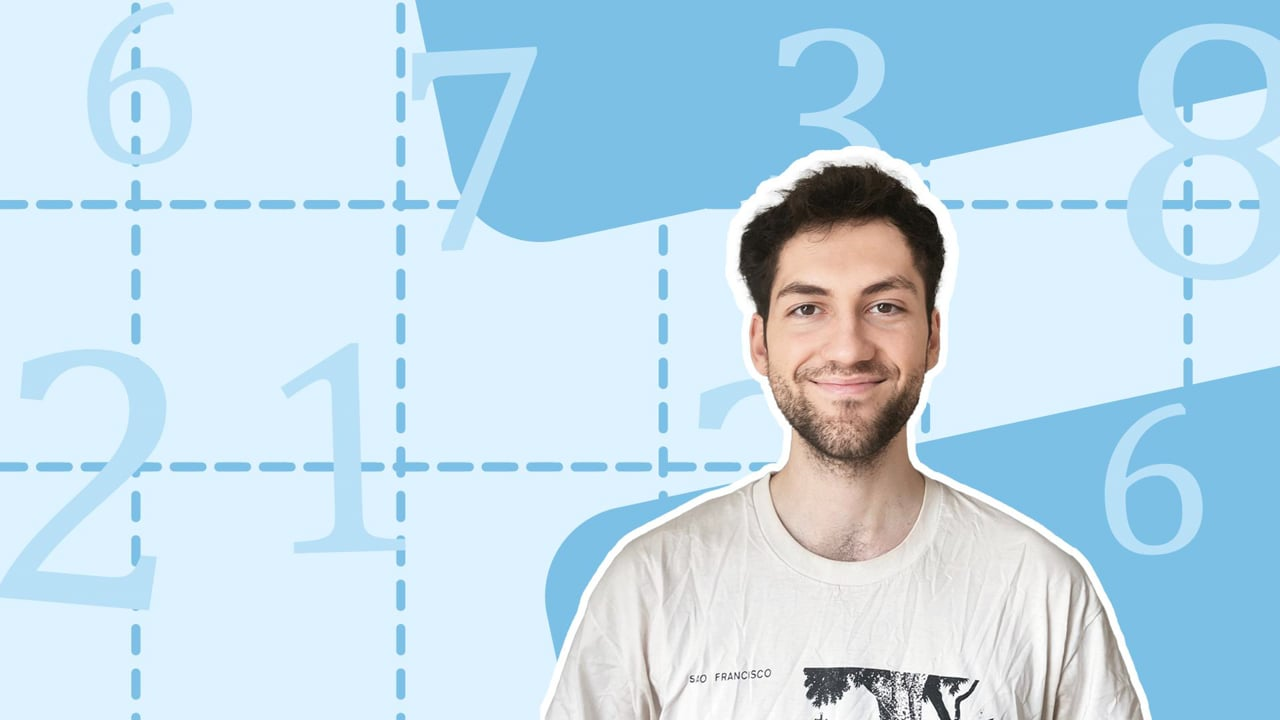Chapter overviewMaths

Number and place value

Multiplication and division

Fractions

Measurement

Geometry - properties of shapes

Statistics

Maths

# Fractions of a set of objects0%

Summary

# Fractions of a set of objects

## In a nutshell

A fraction helps you to describe parts of whole objects.  Fractions have one number on top of another, separated by a fraction line.  They are written using a numerator (top number) and denominator (bottom number).

##### Example 1

A pizza is cut into $6$ equal slices.  One slice is a part of the pizza and there are six parts in total.  What fraction of the pizza is this?

Each part of the pizza here is called a sixth and is written:

$\underline{\dfrac{1}{6}}$​​

## Writing fractions

#### Procedure

 1 Count how many parts there are in total. This will be the denominator. Tip: If the shape has not been split up yet, divide it yourself into equal parts. 2 Count how many parts are highlighted or 'shaded'.  This will be the numerator. 3 Write the numerator on top of the fraction line and the denominator underneath the fraction line.

##### Example 2

The grey section in the following image represents one part.  What fraction of the image is shaded?

Divide the shape into equal parts:

Count how many parts there are in total: $4$

Count how many parts are shaded: $1$

Write the numerator and denominator, separated by the fraction line.

$\underline{\frac{1}{4}}$

FAQs

• Question: What is a denominator?

Answer: The number on the bottom of the fraction line.

• Question: What is a numerator?

Answer: The number on top of the fraction line.

• Question: How do you write out a fraction?

Answer: 1. Count how many parts there are in total. This will be the denominator. 2. Count how many parts are highlighted or 'shaded'. This will be the numerator. 3. Write the numerator on top of the fraction line and the denominator underneath the fraction line.

• Question: What is a fraction?

Answer: A fraction helps you to describe parts of whole objects. Fractions have one number on top of another, separated by a fraction line.

Theory

Exercises